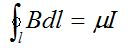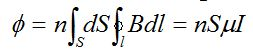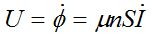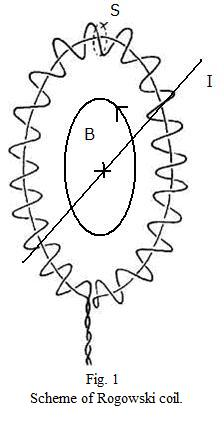Large Rogowski Coil

Principle of Operation

One of means of current measurement is the use of Rogowski coil. This inductive sensor is based on Ampere’s law in its integral form:where I stands for current enveloped by this coil and B stands for magnetic field induced by this current (see fig. 1). Total magnetic flux in Rogowski coil can be expressed as:where n stands for number of turns of coil per unit of length, S is area of a single loop of coil (given that all the turns are assumed to be of same area) and I is measured current. The measured signal at the ends of the coil is in form of voltage U:It is therefore evident that measured voltage has to be integrated and multiplied by a constant in order to obtain the measured quantity (current I). The integration of signals of Rogowski coils on tokamak GOLEM is carried out numerically.

Please note that the wire of the coil does not make a complete turn around the toroidal chamber, instead it is back-wound (see fig. 1). This is necessary, because the coil would otherwise pick also a strong toroidal magnetic field signal.Characterization and Parameters of Sensor

 Large Rogowski coil on tokamak GOLEM serves as sensor of electric current driven through plasma column and conducting chamber. Signal picked up by the coil is a superposition of these two signals, since the coil encircles both the chamber and the plasma. Thus, in order to obtain the information on plasma current, the cross-talk of current in chamber has to be eliminated. The parameters of this coil are summarized in tab. 1.In tab. 1, l stands for length of the coil, Dwire is diameter of the wire of coil and Dinner represents the diameter of turns of coil. n stands for number of turns of coil per unit of length. Finally, K1 stands for constant with which the integrated voltage has to be multiplied with in order to obtain the current flowing through area encircled by this coil. Please note that value of K1 calibration constant was determined by empirical means. Thus the value in tab. 1 differs a little from the value, which would be obtained by analytical means (using geometrical parameters of coil stated in tab. 1).